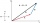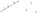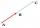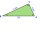# Vector + analytic geometry - math problems

#### Number of problems found: 35

• VectorDetermine coordinates of the vector u=CD if C[19;-7], D[-16,-5].
• Coordinates of vectorDetermine the coordinate of a vector u=CD if C(19;-7) and D(-16;-5)
• Unit vector 2DDetermine coordinates of unit vector to vector AB if A[-6; 8], B[-18; 10].
• Vector sumThe magnitude of the vector u is 12 and the magnitude of the vector v is 8. Angle between vectors is 61°. What is the magnitude of the vector u + v?
• 3d vector componentThe vector u = (3.9, u3) and the length of the vector u is 12. What is is u3?
• Vector perpendicularFind the vector a = (2, y, z) so that a⊥ b and a ⊥ c where b = (-1, 4, 2) and c = (3, -3, -1)
• LineLine p passing through A[-10, 6] and has direction vector v=(3, 2). Is point B[7, 30] on the line p?
• Place vectorPlace the vector AB, if A (3, -1), B (5,3) in the point C (1,3) so that AB = CO
• Vector v4Find the vector v4 perpendicular to vectors v1 = (1, 1, 1, -1), v2 = (1, 1, -1, 1) and v3 = (0, 0, 1, 1)
• Angle of the body diagonalsUsing vector dot product calculate the angle of the body diagonals of the cube.
• Vector - basic operationsThere are given points A [-9; -2] B [2; 16] C [16; -2] and D [12; 18] a. Determine the coordinates of the vectors u=AB v=CD s=DB b. Calculate the sum of the vectors u + v c. Calculate difference of vectors u-v d. Determine the coordinates of the vector w
• Vector equationLet’s v = (1, 2, 1), u = (0, -1, 3) and w = (1, 0, 7) . Solve the vector equation c1 v + c2 u + c3 w = 0 for variables c1 c2, c3 and decide weather v, u and w are linear dependent or independent
• Points collinearShow that the point A(-1,3), B(3,2), C(11,0) are col-linear.
• Linear independenceDetermine if vectors u=(-4; -5) and v=(20; 25) are linear Linear dependent.
• Ascend vs. descentWhich function is growing? a) y = 2-x b) y = 20 c) y = (x + 2). (-5) d) y = x-2
• Three pointsThree points K (-3; 2), L (-1; 4), M (3, -4) are given. Find out: (a) whether the triangle KLM is right b) calculate the length of the line to the k side c) write the coordinates of the vector LM d) write the directional form of the KM side e) write the d
• Coordinates of square verticesThe ABCD square has the center S [−3, −2] and the vertex A [1, −3]. Find the coordinates of the other vertices of the square.
• Angle between vectorsFind the angle between the given vectors to the nearest tenth of a degree. u = (-22, 11) and v = (16, 20)
• Calculate 6Calculate the distance of a point A[0, 2] from a line passing through points B[9, 5] and C[1, -1].
• Parametric formCalculate the distance of point A [2,1] from the line p: X = -1 + 3 t Y = 5-4 t Line p has a parametric form of the line equation. ..

Do you have an interesting mathematical word problem that you can't solve it? Submit a math problem, and we can try to solve it.

We will send a solution to your e-mail address. Solved examples are also published here. Please enter the e-mail correctly and check whether you don't have a full mailbox.

Please do not submit problems from current active competitions such as Mathematical Olympiad, correspondence seminars etc...

For Basic calculations in analytic geometry is a helpful line slope calculator. From coordinates of two points in the plane it calculate slope, normal and parametric line equation(s), slope, directional angle, direction vector, the length of segment, intersections the coordinate axes etc. Our vector sum calculator can add two vectors given by its magnitudes and by included angle. Vector - math word problems. Analytic geometry - math word problems.